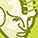# Other Technologies General Forum DiscussionsLevel 1
Level 1# How to modeling/graph Typical performance characteristics current consumption

Hi,

I would like to graph/ modeling typical performance characteristics current consumption (Current consumption Iq versus
output current IOUT and Current consumption Iq versus junction temperature T ). The problem is that in the datasheet ( linear voltage regulator TLS115D0LD) shows the Thermal considerations equations ( power dissipation and thermal resistance) but seems linear while the graphs shows an exponential-like behavior. Is there any specific modeling to obtain the graphs? (see attachment).

Best

1 SolutionModeratorModerator# Re: How to modeling/graph Typical performance characteristics current consumption

Hello,

Thank you for posting in Infineon community,

The objective of Power dissipation and Thermal resistance Equations shown in "5.3 Thermal considerations" is to estimate the maximum Power dissipation and maximum thermal resistance allowable for a given lout (max), Vout, and Vin in order to be within max thermal limits (Tj).
Pd equation is still non-linear w.r.t Iout if you substitute Iq with the exponential curve from Iq vs Iout.
But as mentioned Iq can be derived from Iq vs Iout (exponential curve) for a given Iout(fixed) and estimate Pd.

Thanks

AnilModeratorModerator# Re: How to modeling/graph Typical performance characteristics current consumption

Hello,

Thank you for posting in Infineon community,

The objective of Power dissipation and Thermal resistance Equations shown in "5.3 Thermal considerations" is to estimate the maximum Power dissipation and maximum thermal resistance allowable for a given lout (max), Vout, and Vin in order to be within max thermal limits (Tj).
Pd equation is still non-linear w.r.t Iout if you substitute Iq with the exponential curve from Iq vs Iout.
But as mentioned Iq can be derived from Iq vs Iout (exponential curve) for a given Iout(fixed) and estimate Pd.

Thanks

Anil